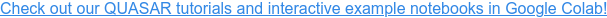QUASAR Examples

# QUASAR: Stochastic Programming in Scala

In this blog post, I am going to show you just how easy it is to set up and solve a stochastic programming problem. In the previous blog post, we have used our Python interface. In this example, I am going to call our Java API from Scala, which is another popular programming language for data science.

In :
``` import \$ivy.`org.plotly-scala::plotly-almond:0.8.1`
import com.quantego.quasar.model._, com.quantego.quasar.optimizer._, com.quantego.quasar.stochastic.process._
import plotly.Scatter, plotly.Almond, plotly.Box, plotly.element._, plotly.layout._
```

### Data and Parameters¶

The decision problem that guides this example is a simple storage optimization model. The decision-maker must decide when and how much to inject and widthdraw from a storage. What makes the decision problem stochastic is that prices follow some random process and any decision taken now effects future opportunities to inject and withdraw as well as how profitable the storage will be. We are planning our little storage over next 12 time periods (think of months) and need to account for capacity limits as well as possibly losses.

In :
``` val timeHorizon = 12
val loss = 0.1
val maxFill = 100
val maxRate = 25
```

### Model Formulation¶

What is special about QUASAR is that you can formulate any stochastic programming problem just like it was a linear program, but unlike with conventional solvers, any model parameter can be a random variable. All it takes is passing the name of the parameter as a string that must be unique for a given stage.

In :
``` // decision model
val model = new DecisionProblem()
// starting state
var s = Map(-1 -> model.addVariable(0).lb(25).ub(25))
// multiple stages
var obj = new Expression()
for (t <- 0 until timeHorizon) {
// decision variables
s += (t -> model.addVariable(t, "storage").ub(maxFill))
// balance constraint
// objective with random variable
}
model.maximize(obj)
```

### Time Series Data¶

In the above example, prices are labeled as random variables. Now we need to define a stochastic process that describes how prices evolve ofer time. If you happen to have a sample of price scenarios, for example from another model or from real data, you can simply import those as time series samples.

In :
``` val ts = TimeSeriesSample.createSampleFromCSV("prices.csv")
```

### Start the Solver¶

QUASAR's solvers can take it from here. Just pass the model and the time series sample, and the dynamic optimizer will automatically decompose the problem, generate a scenario lattice, and solve it until the solution has converged. This 12-stage problem does not even take a second to be solved.

In :
``` // optimize problem
val opt = DynamicOptimizerBuilder.create(model, ts, 100).build()
opt.solve()
```

```[17:30:31 INFO] Lattice: Generating diffusion lattice...
[17:30:31 INFO] Lattice: Removing empty and hanging nodes...
[17:30:31 INFO] Lattice: Finalizing lattice...
[17:30:31 INFO] Lattice: The final lattice has 12 stages, 1101 nodes with 1 root node(s) and 100 leaf nodes, and 1100 arcs.
[17:30:32 INFO] DynamicOptimizer: Starting optimization...
[17:30:32 INFO] DynamicOptimizer: solver=CLP | forward pass=SINGLEPASS | risk measure=EXPECTATION | integer cuts=RELAXED
[17:30:32 INFO]       iter	exp reward	sim reward	 std error	   size	   seconds	 cut count	LPs solved	 primal Dt	   dual Dt
[17:30:32 INFO]          5	    754.26	    602.19	     45.67	      5	      0.07	      4722	      5620	       NaN	       NaN
[17:30:32 INFO]         10	    701.20	    450.20	    173.61	     10	      0.10	      7022	     11240	      0.00	      0.00
[17:30:32 INFO]         15	    699.10	    487.67	    115.47	     15	      0.13	      7649	     16860	      0.00	      0.02
[17:30:32 INFO]         20	    693.72	    521.29	     87.88	     20	      0.16	      8776	     22480	      0.00	      0.06
[17:30:32 INFO]         25	    692.15	    532.56	     88.10	     20	      0.19	      9337	     28100	      0.00	      0.06
[17:30:32 INFO]         30	    687.60	    641.01	     28.23	     20	      0.22	     10305	     33720	      0.00	      0.06
[17:30:32 INFO]         35	    686.63	    654.67	     25.79	     20	      0.24	     10716	     39340	      0.00	      0.01
[17:30:32 INFO]         40	    686.62	    649.48	     24.17	     20	      0.27	     10955	     44960	      0.00	      0.01
[17:30:32 INFO]         45	    685.18	    689.28	     36.29	     20	      0.29	     11412	     50580	      0.00	      0.03
[17:30:32 INFO]         50	    685.08	    657.57	     33.15	     20	      0.32	     11536	     56200	      0.00	      0.05
[17:30:32 INFO]         55	    684.85	    689.82	     35.02	     20	      0.34	     11726	     61820	      0.00	      0.05
[17:30:32 INFO]         60	    684.55	    682.10	     14.29	    100	      0.36	     11947	     67440	      0.00	      0.01
[17:30:32 INFO] DynamicOptimizer: STATUS=BOUNDS_CONVERGED
```

### Inspect the Solution¶

The solution of a stochastic program is a decision policy which is itself stochastic (or, more precisely, a function of the scenario as it unfolds over time). The best way to inspect the decision policy is by simulating the optimal policy and visulize the distribution of decision variables and objective value. In this way, we gain insights into the distribution of decision variables, objective values, and their covariance with the random process.

In :
``` // simulate decision process
val nSim = 100
val sim = opt.getPolicy.simulate(nSim)
```

```[17:30:32 INFO] Policy: Starting 100 forward simulations...
[17:30:32 INFO] Policy: Simulated total reward: avg=689.26, stdev=141.35, 95%-CI=28.04
```

For example, we can use Plotly to obtain a box plot of the simulated objective values at each stage.

In :
``` Almond.plot(
Seq.tabulate(timeHorizon)(
i => Box(
Seq.tabulate(nSim)(
j => sim.getReward(j,i)
),
line=Line(color=Color.RGBA(31,119,180,1.0), width=1),
)
),
Layout(
showlegend=false
)
)
```

Or we can visualize scenarios of possible storage fill levels over the entire time horizon by means of a spaghetti chart.

In :
``` Almond.plot(
Seq.tabulate(nSim)(
i => Scatter(
Seq.tabulate(timeHorizon)(
j => sim.getDecision(i,j,"storage")
),
mode = ScatterMode(ScatterMode.Lines),
line = Line(color=Color.RGBA(31,119,180,0.1)),
hoverinfo = HoverInfo.Skip
)
),
Layout(
showlegend=false
)
)
```

Of course, this little example just scratches the surface of what can be done with the solver, and there are many more features to explore. If you want to see other examples, check out some of our Colab notebooks, which allow you to run QUASAR right in your browser.